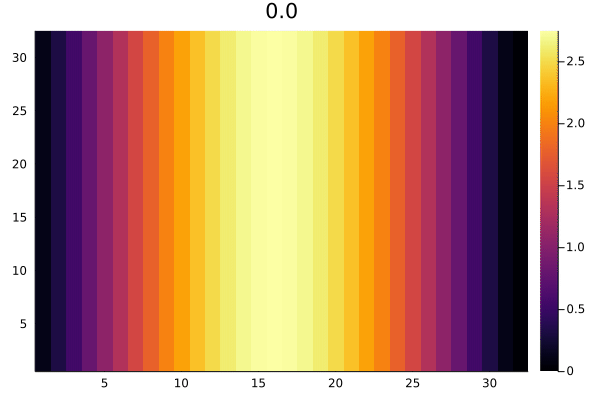# Tutorial

## Using the Brusselator PDE as an example

The Brusselator PDE is defined as follows:

\begin{align} \frac{\partial u}{\partial t} &= 1 + u^2v - 4.4u + \alpha(\frac{\partial^2 u}{\partial x^2} + \frac{\partial^2 u}{\partial y^2}) + f(x, y, t)\\ \frac{\partial v}{\partial t} &= 3.4u - u^2v + \alpha(\frac{\partial^2 v}{\partial x^2} + \frac{\partial^2 v}{\partial y^2}) \end{align}

where

$$$f(x, y, t) = \begin{cases} 5 & \quad \text{if } (x-0.3)^2+(y-0.6)^2 ≤ 0.1^2 \text{ and } t ≥ 1.1 \\ 0 & \quad \text{else} \end{cases}$$$

and the initial conditions are

\begin{align} u(x, y, 0) &= 22\cdot (y(1-y))^{3/2} \\ v(x, y, 0) &= 27\cdot (x(1-x))^{3/2} \end{align}

with the periodic boundary condition

\begin{align} u(x+1,y,t) &= u(x,y,t) \\ u(x,y+1,t) &= u(x,y,t) \end{align}

on a timespan of $t \in [0,11.5]$.

## Solving with MethodOfLines

With ModelingToolkit.jl, we first symbolicaly define the system, see also the docs for PDESystem:

using ModelingToolkit, MethodOfLines, OrdinaryDiffEq, DomainSets

@parameters x y t
@variables u(..) v(..)
Dt = Differential(t)
Dx = Differential(x)
Dy = Differential(y)
Dxx = Differential(x)^2
Dyy = Differential(y)^2

∇²(u) = Dxx(u) + Dyy(u)

brusselator_f(x, y, t) = (((x-0.3)^2 + (y-0.6)^2) <= 0.1^2) * (t >= 1.1) * 5.

x_min = y_min = t_min = 0.0
x_max = y_max = 1.0
t_max = 11.5

α = 10.

u0(x,y,t) = 22(y*(1-y))^(3/2)
v0(x,y,t) = 27(x*(1-x))^(3/2)

eq = [Dt(u(x,y,t)) ~ 1. + v(x,y,t)*u(x,y,t)^2 - 4.4*u(x,y,t) + α*∇²(u(x,y,t)) + brusselator_f(x, y, t),
Dt(v(x,y,t)) ~ 3.4*u(x,y,t) - v(x,y,t)*u(x,y,t)^2 + α*∇²(v(x,y,t))]

domains = [x ∈ Interval(x_min, x_max),
y ∈ Interval(y_min, y_max),
t ∈ Interval(t_min, t_max)]

# Periodic BCs
bcs = [u(x,y,0) ~ u0(x,y,0),
u(0,y,t) ~ u(1,y,t),
u(x,0,t) ~ u(x,1,t),

v(x,y,0) ~ v0(x,y,0),
v(0,y,t) ~ v(1,y,t),
v(x,0,t) ~ v(x,1,t)]

@named pdesys = PDESystem(eq,bcs,domains,[x,y,t],[u(x,y,t),v(x,y,t)])

For a list of limitations constraining which systems will work, see here

## Method of lines discretization

Then, we create the discretization, leaving the time dimension undiscretized by supplying t as an argument. Optionally, all dimensions can be discretized in this step, just remove the argument t and supply t=>dt in the dxs. See here for more information on the MOLFiniteDifference constructor arguments and options.

N = 32

dx = (x_max-x_min)/N
dy = (y_max-y_min)/N

order = 2

discretization = MOLFiniteDifference([x=>dx, y=>dy], t, approx_order=order, grid_align=center_align)

Next, we discretize the system, converting the PDESystem in to an ODEProblem or NonlinearProblem.

# Convert the PDE problem into an ODE problem
println("Discretization:")
@time prob = discretize(pdesys,discretization)

## Solving the problem

Now your problem can be solved with an appropriate ODE solver, or Nonlinear solver if you have not supplied a time dimension in the MOLFiniteDifference constructor. Include these solvers with using OrdinaryDiffEq or using NonlinearSolve, then call sol = solve(prob, AppropriateSolver()) or sol = NonlinearSolve.solve(prob, AppropriateSolver()). For more information on the available solvers, see the docs for DifferentialEquations.jl, NonlinearSolve.jl and SteadyStateDiffEq.jl. Tsit5() is a good first choice of solver for many problems.

println("Solve:")
@time sol = solve(prob, TRBDF2(), saveat=0.1)

## Extracting results

To retrieve your solution, for example for u, use sol[u]. To get the time axis, use sol.t.

Due to current limitations in the sol interface, above 1 discretized dimension the result must be manually reshaped to correctly display the result, best done with the help of the get_discrete helper function. Here is an example of how to do this:

grid = get_discrete(pdesys, discretization)
discrete_x = grid[x]
discrete_y = grid[y]
discrete_t = sol[t]

solu = [map(d -> sol[d][i], grid[u(x, y, t)]) for i in 1:length(sol[t])]
solv = [map(d -> sol[d][i], grid[v(x, y, t)]) for i in 1:length(sol[t])]

The result after plotting an animation:

For u:

using Plots
anim = @animate for k in 1:length(discrete_t)
heatmap(solu[k][2:end, 2:end], title="$(discrete_t[k])") # 2:end since end = 1, periodic condition end gif(anim, "plots/Brusselator2Dsol_u.gif", fps = 8)For v: anim = @animate for k in 1:length(discrete_t) heatmap(solv[k][2:end, 2:end], title="$(discrete_t[k])")
end
gif(anim, "plots/Brusselator2Dsol_v.gif", fps = 8)